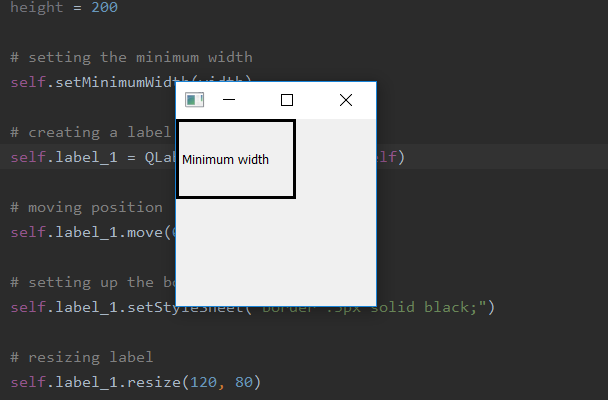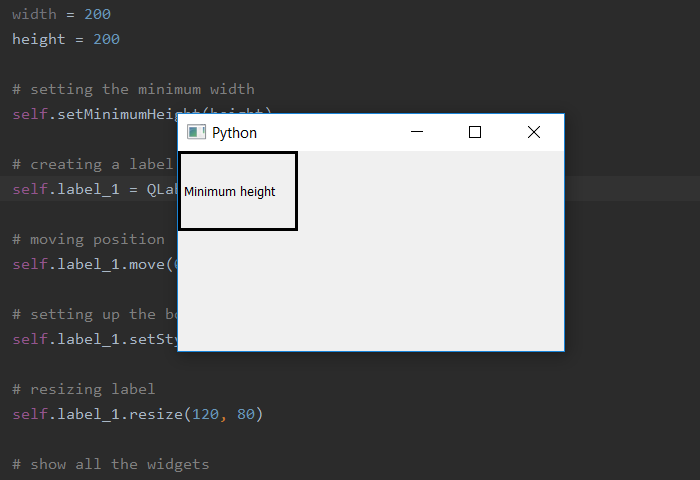# PyQt5 – Set minimum window size | setMinimumWidth and setMinimumHeight method

• Last Updated : 26 Mar, 2020

When we create a window, by default the window size is resizable although, we can use setMinimumSize() method to set the minimum size of the window. But what if we want to set minimum length only for width or height only, in order to do so we use `setMinimumWidth()` method to set minimum width and `setMinimumHeight()` method to set minimum height. When we use these method other length will be variable i.e there will be no minimum length to it, it can shrinks as much it can.

Syntax :

Attention geek! Strengthen your foundations with the Python Programming Foundation Course and learn the basics.

To begin with, your interview preparations Enhance your Data Structures concepts with the Python DS Course. And to begin with your Machine Learning Journey, join the Machine Learning - Basic Level Course

```self.setMinimumWidth(width)
self.setMinimumHeight(height)
```

Argument : Both takes integer as argument.

Action performed.
`setMinimumWidth()` sets the minimum width.
`setMinimumHeight()` sets the minimum height.

Code for setting minimum width :

 `# importing the required libraries`` ` `from` `PyQt5.QtCore ``import` `*` `from` `PyQt5.QtGui ``import` `*` `from` `PyQt5.QtWidgets ``import` `*` `import` `sys`` ` ` ` `class` `Window(QMainWindow):``    ``def` `__init__(``self``):``        ``super``().__init__()`` ` ` ` `        ``# set the title``        ``self``.setWindowTitle(``"Python"``)`` ` `        ``width ``=` `200``        ``height ``=` `200`` ` `        ``# setting the minimum width``        ``self``.setMinimumWidth(width)`` ` `        ``# creating a label widget``        ``self``.label_1 ``=` `QLabel(``"Minimum width"``, ``self``)`` ` `        ``# moving position``        ``self``.label_1.move(``0``, ``0``)`` ` `        ``# setting up the border``        ``self``.label_1.setStyleSheet(``"border :3px solid black;"``)`` ` `        ``# resizing label``        ``self``.label_1.resize(``120``, ``80``)`` ` `        ``# show all the widgets``        ``self``.show()`` ` ` ` `# create pyqt5 app``App ``=` `QApplication(sys.argv)`` ` `# create the instance of our Window``window ``=` `Window()`` ` `# start the app``sys.exit(App.``exec``())`

Output :Code for setting minimum width :

 `# importing the required libraries`` ` `from` `PyQt5.QtCore ``import` `*` `from` `PyQt5.QtGui ``import` `*` `from` `PyQt5.QtWidgets ``import` `*` `import` `sys`` ` ` ` `class` `Window(QMainWindow):``    ``def` `__init__(``self``):``        ``super``().__init__()`` ` ` ` `        ``# set the title``        ``self``.setWindowTitle(``"Python"``)`` ` `        ``width ``=` `200``        ``height ``=` `200`` ` `        ``# setting the minimum width``        ``self``.setMinimumHeight(height)`` ` `        ``# creating a label widget``        ``self``.label_1 ``=` `QLabel(``"Minimum height"``, ``self``)`` ` `        ``# moving position``        ``self``.label_1.move(``0``, ``0``)`` ` `        ``# setting up the border``        ``self``.label_1.setStyleSheet(``"border :3px solid black;"``)`` ` `        ``# resizing label``        ``self``.label_1.resize(``120``, ``80``)`` ` `        ``# show all the widgets``        ``self``.show()`` ` ` ` `# create pyqt5 app``App ``=` `QApplication(sys.argv)`` ` `# create the instance of our Window``window ``=` `Window()`` ` `# start the app``sys.exit(App.``exec``())`

Output :My Personal Notes arrow_drop_up